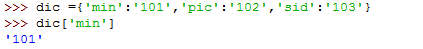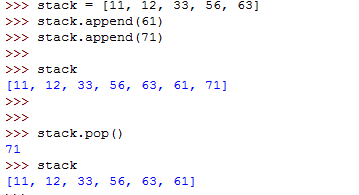# Programming Python 101: Data Structures

Well, in the last blog post, we started off with the python programming. This post is all about data structures, so it would be bit precise and short.

As we have discussed about Arrays in our last blog post, I would like to extend it to other data structures:

1. Dictionary:

Defining dictionary is as simple as defining variables in python, you just need to write code as follows:In dictionary, the first one is the key to access the value associated with key. So, if we want to find a particular value associated with a key we access them directly by calling the key in the dictionary.

2. Stacks:

In python, lists represents all the data structures, so, similarly, we can implement Stacks. We have push and pop operations for stacks, and as we know that in stacks, the storage is done on basis of LIFO concept. So, let’s see, how we can implement stacks in Python:|Here, in Python, PUSH operation is performed by .append(‘argument’)  method, where we can add any element at the top of the stack. And POP operation performed by .pop() which pops the element at the top and prints the popped element. To print the values in the stack, just type the stack name and then enter, stack will be printed.

3. Queue:

Queues are similarly implemented by using Stacks, but we need use collections.deque to implement fast append and pop at both the ends. This is because in Queue, we follow concept of FIFO to store and pop elements. So, the code is written as follows:As similar to Stack, data is inserted in Queue by using .append(‘argument’) , but for popping elements, we use method: .popleft() which prints the popped element and removes it from the queue.

This is all for today’s tutorial, I hopes that this tutorial will help in learning a bit of Python. Meet you with my next blog on Python.